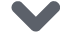# 使用 PyTorch 进行 风格迁移（Neural-Transfer）

### 3.导包并选择设备

• torch, torch.nn, numpy使用PyTorch进行风格转换必不可少的包

• torch.optim高效的梯度下降

• PIL, PIL.Image, matplotlib.pyplot加载和展示图片

• torchvision.transforms将PIL图片转换成张量

• torchvision.models训练或加载预训练模型

• copy对模型进行深度拷贝；系统包

from __future__ import print_functionimport torchimport torch.nn as nnimport torch.nn.functional as Fimport torch.optim as optimfrom PIL import Imageimport matplotlib.pyplot as pltimport torchvision.transforms as transformsimport torchvision.models as modelsimport copy

torch.device .to(device)方法也被用来将张量或者模型移动到指定设备。

device = torch.device("cuda" if torch.cuda.is_available() else "cpu")

### 4.加载图片

images文件夹。

# 所需的输出图像大小imsize = 512 if torch.cuda.is_available() else 128  # use small size if no gpuloader = transforms.Compose([    transforms.Resize(imsize),  # scale imported image    transforms.ToTensor()])  # transform it into a torch tensordef image_loader(image_name):    image = Image.open(image_name)    # fake batch dimension required to fit network's input dimensions    image = loader(image).unsqueeze(0)    return image.to(device, torch.float)style_img = image_loader("./data/images/neural-style/picasso.jpg")content_img = image_loader("./data/images/neural-style/dancing.jpg")assert style_img.size() == content_img.size(),     "we need to import style and content images of the same size"现在，让我们创建一个方法，通过重新将图片转换成PIL格式来展示，并使用plt.imshow展示它的拷贝。我们将尝试展示内容和风格图片来确保它们被正确的导入。unloader = transforms.ToPILImage()  # reconvert into PIL imageplt.ion()def imshow(tensor, title=None):    image = tensor.cpu().clone()  # we clone the tensor to not do changes on it    image = image.squeeze(0)      # remove the fake batch dimension    image = unloader(image)    plt.imshow(image)    if title is not None:        plt.title(title)    plt.pause(0.001) # pause a bit so that plots are updatedplt.figure()imshow(style_img, title='Style Image')plt.figure()imshow(content_img, title='Content Image')

### 5.损失函数

#### 5.1 内容损失

C之间的加权内容间距W_CL*D_C^L(X,C)该方法必须知道内容图片（F_CL）的特征映射来计算内容间距。我们使用一个以F_CL作为

class ContentLoss(nn.Module):    def __init__(self, target,):        super(ContentLoss, self).__init__()        # 我们从用于动态计算梯度的树中“分离”目标内容：        # 这是一个声明的值，而不是变量。         # 否则标准的正向方法将引发错误。        self.target = target.detach()    def forward(self, input):        self.loss = F.mse_loss(input, self.target)        return input

#### 5.2 风格损失

F_XL被重塑成F̂_XL，一个 KxN的矩阵，其中K是L层特征映射的数量，N是任何向量化特征映射F_XL^K的长度。例如，第一行的F̂_XL

def gram_matrix(input):    a, b, c, d = input.size()  # a=batch size(=1)    # 特征映射 b=number    # (c,d)=dimensions of a f. map (N=c*d)    features = input.view(a * b, c * d)  # resise F_XL into hat F_XL    G = torch.mm(features, features.t())  # compute the gram product    # 我们通过除以每个特征映射中的元素数来“标准化”gram矩阵的值.    return G.div(a * b * c * d)

class StyleLoss(nn.Module):    def __init__(self, target_feature):        super(StyleLoss, self).__init__()        self.target = gram_matrix(target_feature).detach()    def forward(self, input):        G = gram_matrix(input)        self.loss = F.mse_loss(G, self.target)        return input

### 6.导入模型

PyTorch 的 VGG 模型实现被分为了两个字 Sequential 模型：features（包含卷积层和池化层）和classifier（包含全连接层）。

cnn = models.vgg19(pretrained=True).features.to(device).eval()

cnn_normalization_mean = torch.tensor([0.485, 0.456, 0.406]).to(device)cnn_normalization_std = torch.tensor([0.229, 0.224, 0.225]).to(device)# 创建一个模块来规范化输入图像# 这样我们就可以轻松地将它放入nn.Sequential中class Normalization(nn.Module):    def __init__(self, mean, std):        super(Normalization, self).__init__()        # .view the mean and std to make them [C x 1 x 1] so that they can        # directly work with image Tensor of shape [B x C x H x W].        # B is batch size. C is number of channels. H is height and W is width.        self.mean = torch.tensor(mean).view(-1, 1, 1)        self.std = torch.tensor(std).view(-1, 1, 1)    def forward(self, img):        # normalize img        return (img - self.mean) / self.std

# 期望的深度层来计算样式/内容损失：content_layers_default = ['conv_4']style_layers_default = ['conv_1', 'conv_2', 'conv_3', 'conv_4', 'conv_5']def get_style_model_and_losses(cnn, normalization_mean, normalization_std,                               style_img, content_img,                               content_layers=content_layers_default,                               style_layers=style_layers_default):    cnn = copy.deepcopy(cnn)    # 规范化模块    normalization = Normalization(normalization_mean, normalization_std).to(device)    # 只是为了拥有可迭代的访问权限或列出内容/系统损失    content_losses = []    style_losses = []    # 假设cnn是一个nn.Sequential，    # 所以我们创建一个新的nn.Sequential来放入应该按顺序激活的模块    model = nn.Sequential(normalization)    i = 0  # increment every time we see a conv    for layer in cnn.children():        if isinstance(layer, nn.Conv2d):            i += 1            name = 'conv_{}'.format(i)        elif isinstance(layer, nn.ReLU):            name = 'relu_{}'.format(i)            # 对于我们在下面插入的ContentLoss和StyleLoss，            # 本地版本不能很好地发挥作用。所以我们在这里替换不合适的            layer = nn.ReLU(inplace=False)        elif isinstance(layer, nn.MaxPool2d):            name = 'pool_{}'.format(i)        elif isinstance(layer, nn.BatchNorm2d):            name = 'bn_{}'.format(i)        else:            raise RuntimeError('Unrecognized layer: {}'.format(layer.__class__.__name__))        model.add_module(name, layer)        if name in content_layers:            # 加入内容损失:            target = model(content_img).detach()            content_loss = ContentLoss(target)            model.add_module("content_loss_{}".format(i), content_loss)            content_losses.append(content_loss)        if name in style_layers:            # 加入风格损失:            target_feature = model(style_img).detach()            style_loss = StyleLoss(target_feature)            model.add_module("style_loss_{}".format(i), style_loss)            style_losses.append(style_loss)    # 现在我们在最后的内容和风格损失之后剪掉了图层    for i in range(len(model) - 1, -1, -1):        if isinstance(model[i], ContentLoss) or isinstance(model[i], StyleLoss):            break    model = model[:(i + 1)]    return model, style_losses, content_losses

input_img = content_img.clone()# 如果您想使用白噪声而取消注释以下行：# input_img = torch.randn(content_img.data.size(), device=device)# 将原始输入图像添加到图中：plt.figure()imshow(input_img, title='Input Image')

### 7.梯度下降

def get_input_optimizer(input_img):    # 此行显示输入是需要渐变的参数    optimizer = optim.LBFGS([input_img.requires_grad_()])    return optimizer

def run_style_transfer(cnn, normalization_mean, normalization_std,                       content_img, style_img, input_img, num_steps=300,                       style_weight=1000000, content_weight=1):    """Run the style transfer."""    print('Building the style transfer model..')    model, style_losses, content_losses = get_style_model_and_losses(cnn,        normalization_mean, normalization_std, style_img, content_img)    optimizer = get_input_optimizer(input_img)    print('Optimizing..')    run =     while run <= num_steps:        def closure():            # 更正更新的输入图像的值            input_img.data.clamp_(0, 1)            optimizer.zero_grad()            model(input_img)            style_score = 0            content_score = 0            for sl in style_losses:                style_score += sl.loss            for cl in content_losses:                content_score += cl.loss            style_score *= style_weight            content_score *= content_weight            loss = style_score + content_score            loss.backward()            run += 1            if run % 50 == 0:                print("run {}:".format(run))                print('Style Loss : {:4f} Content Loss: {:4f}'.format(                    style_score.item(), content_score.item()))                print()            return style_score + content_score        optimizer.step(closure)    # 最后的修正......    input_img.data.clamp_(0, 1)    return input_img

output = run_style_transfer(cnn, cnn_normalization_mean, cnn_normalization_std,                            content_img, style_img, input_img)plt.figure()imshow(output, title='Output Image')# sphinx_gallery_thumbnail_number = 4plt.ioff()plt.show()
• 输出结果

Building the style transfer model..Optimizing..run :Style Loss : 4.169304 Content Loss: 4.235329run :Style Loss : 1.145476 Content Loss: 3.039176run :Style Loss : 0.716769 Content Loss: 2.663749run :Style Loss : 0.476047 Content Loss: 2.500893run :Style Loss : 0.347092 Content Loss: 2.410895run :Style Loss : 0.263698 Content Loss: 2.358449

PyTorch 官方60分钟入门教程的配套视频教程：

https://www.bilibili.com/video/av66421076/点击下方 |  | 了解更多